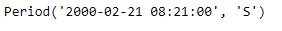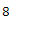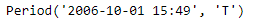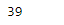GeeksforGeeks App
Open AppBrowser
Continue

# Python | Pandas Period.weekofyear

Python is a great language for doing data analysis, primarily because of the fantastic ecosystem of data-centric python packages. Pandas is one of those packages and makes importing and analyzing data much easier.
Pandas Period.weekofyear attribute return an integer value which is the week number of the year for the given Period object.

Syntax : Period.weekofyear
Parameters : None
Return : week of the year

Example #1: Use Period.weekofyear attribute to find the week number for the given Period object.

## Python3

 `# importing pandas as pd``import` `pandas as pd` `# Create the Period object``prd ``=` `pd.Period(freq ``=``'S'``, year ``=` `2000``, month ``=` `2``,``                  ``day ``=` `21``, hour ``=` `8``, minute ``=` `21``)` `# Print the Period object``print``(prd)`

Output :Now we will use the Period.weekofyear attribute to find the week number of the year

## Python3

 `# return week number for the year``prd.weekofyear`

Output :As we can see in the output, the Period.weekofyear attribute has returned 8 indicating that the period in the prd object lies in the 8th week of the year.
Example #2: Use Period.weekofyear attribute to find the week number for the given Period object.

## Python3

 `# importing pandas as pd``import` `pandas as pd` `# Create the Period object``prd ``=` `pd.Period(freq ``=``'T'``, year ``=` `2006``, month ``=` `10``,``                            ``hour ``=` `15``, minute ``=` `49``)` `# Print the Period object``print``(prd)`

Output :Now we will use the Period.weekofyear attribute to find the week number of the year

## Python3

 `# return week number for the year``prd.weekofyear`

Output :As we can see in the output, the Period.weekofyear attribute has returned 39 indicating that the period in the prd object lies in the 39th week of the year.

My Personal Notes arrow_drop_up